# Main Wind-Force Resisting Systems MWFRS

Wind loads for the design of the main wind-force resisting system are illustrated and specified in the following figures, tables, and formulas. A plus (+) sign denotes pressure toward a surface and a minus (-)sign denotes pressure away from a surface. What is MWFRS? Here we will explain.

## MWFRS Main Wind-Force Resisting Systems for Buidling

External pressure coefficients (Cp) are given in Tables VIII and IX. Figure 1 designates building geometry, and Figure 2 illustrates design wind pressure.
Design Wind Pressures (p) for buildings with height/least horizontal dimension > 4 and f > 1.0 cycle/second, design wind pressures shall be as follows: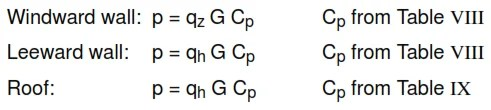qz for windward wall at height Z above ground.

qh for leeward wall at mean roof height Obtain.

qz or qh from Table VI (exposure C) or Table VII (exposure D).

G = 0.85 for exposure C or D.

For flexible buildings or buildings with height/least horizontal dimension > 4, or the natural frequency, f < 1.0 cycle/second, design wind pressure shall be in accordance with ASCE 7-95, including appendix and commentary.

Figure 1 – Building Geometry Designation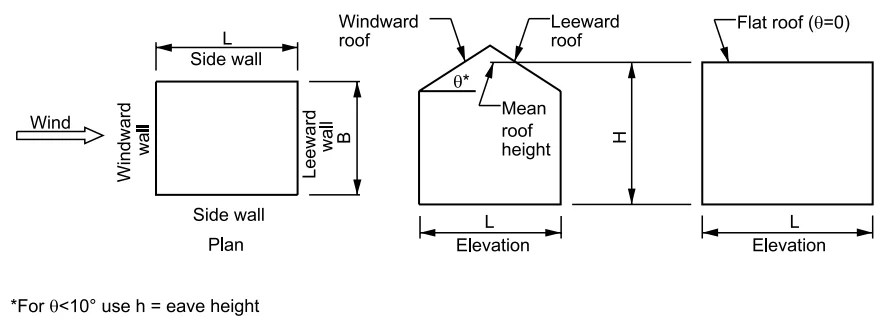Figure 1 – Building Geometry Designation

Figure 2 – Design Wind Pressures Cp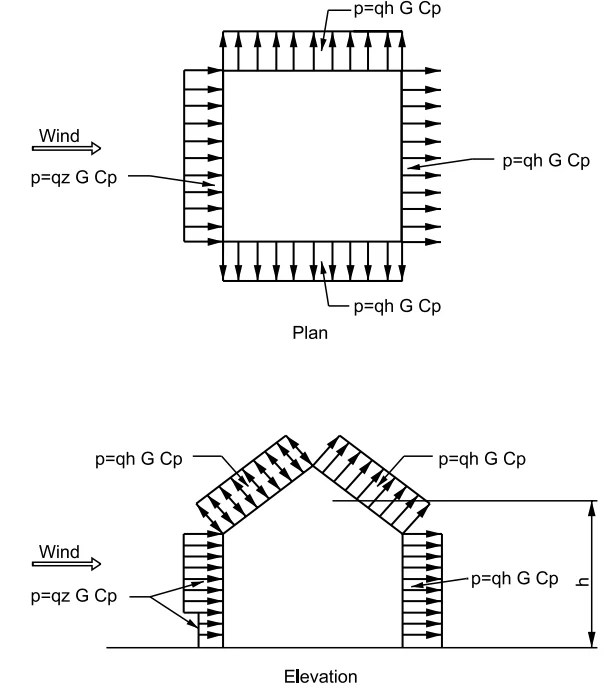Figure 2 – Design Wind Pressures Cp

Table VIII – Wall Pressure Coefficient, Cp Design Wind Pressures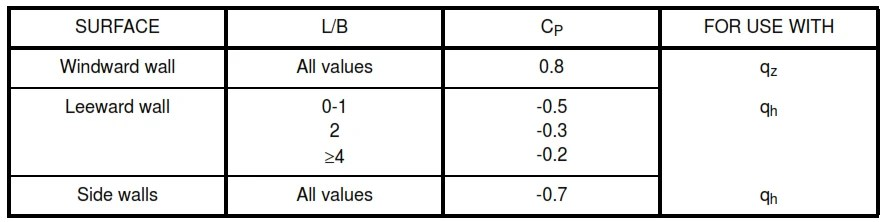Table VIII – Wall Pressure Coefficient, Cp Design Wind Pressures

Notes:
B = Horizontal dimension of building, in m (ft), measured normal to wind direction.
L = Horizontal dimension of building, in m (ft), measured parallel to wind direction.

Table IX – Roof Pressure Coefficient, Cp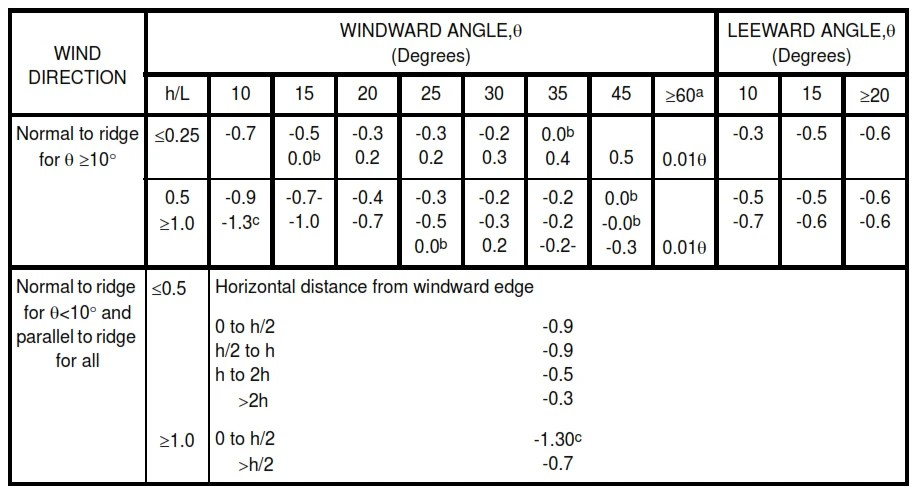Table IX – Roof Pressure Coefficient, Cp

Note:
L = Horizontal dimension of building, in m (ft), measured parallel to wind direction.
h = Mean roof height in m (ft), except that eaves height shall be used for Ѳ ≤ 10 degree

Ѳ = Angle of plane or roof from horizontal, in degrees.
a – For roof slopes greater than 80 degree, use Cp = 0.8.
b – Value is provided for interpolation purpose.
c – Value can be reduced linearly with area over which it is applicable as follows:Plus and minus signs signify pressures acting toward and away from the surface respectively.
Linear interpolation is permitted for values of L/B, h/L, and * other than shown. Interpolation shall only be carried out between values of the same sign. Where no value of the same sign is given, assume 0.0 for interpolation purposes.

When two values of Cp are listed, this indicates that the windward roof slope is subjected to either positive or negative pressure and the roof structure shall be designed for both conditions. Interpolation for intermediate ratio of h/L in this case shall only be carried out between Cp values of like sign. For monoslope roofs, entire roof surface is either a windward or leeward surface.